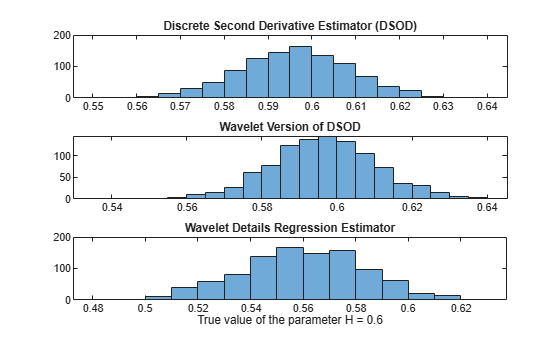# wfbmesti

Parameter estimation of fractional Brownian motion

## Syntax

```HEST = wfbmesti(X) ```

## Description

`HEST = wfbmesti(X)` returns a one-by-three vector `HEST` which contains three estimates of the fractal index `H` of the input signal `X`. The signal `X` is assumed to be a realization of fractional Brownian motion with Hurst index `H`.

The first two elements of the vector are estimates based on the second derivative with the second computed in the wavelet domain.

The third estimate is based on the linear regression in loglog plot, of the variance of detail versus level.

A fractional Brownian motion (`fBm`) is a continuous-time Gaussian process depending on the so-called Hurst parameter ```0 < H < 1```. It generalizes the ordinary Brownian motion corresponding to `H = 0.5` and whose derivative is the white noise. The `fBm` is self-similar in distribution and the variance of the increments is

```Var(fBm(t)-fBm(s)) = v |t-s|^(2H) ```

where `v` is a positive constant.

This special form of the variance of the increments suggests various ways to estimate the parameter `H`. One can find in Bardet et al. a survey of such methods. The `wfbmesti` file provides three different estimates. The first one, due to Istas and Lang, is based on the discrete second-order derivative. The second one is a wavelet-based adaptation and has similar properties. The third one, proposed by Flandrin, estimates `H` using the slope of the loglog plot of the detail variance versus the level. A more recent extension can be found in Abry et al.

## Examples

collapse all

This example shows how to estimate the Hurst index of a fractional Brownian motion. The example simulates 1,000 realizations of fractional Brownian motion with H=0.6. Each realization consists of 10,000 samples. At the end of the simulation, the three estimates of the Hurst index are compared.

Initialize the random number generator for repeatable results. Set the Hurst index equal to 0.6 and the length of the realizations to be 10,000.

```rng default; H = 0.6; len = 10000;```

Generate 1,000 realizations of fractional Brownian motion and compute the estimates of the Hurst parameter.

```n = 1000; Hest = zeros(n,3); for ii = 1:n fBm06 = wfbm(H,len); Hest(ii,:) = wfbmesti(fBm06); end```

Compare the estimates.

```subplot(311), histogram(Hest(:,1)); title('Discrete second derivative estimator (DSOD)') subplot(312), histogram(Hest(:,2)); title('Wavelet version of DSOD') subplot(313), histogram(Hest(:,3)); title('Wavelet details regression estimator') xlabel('True value of the parameter H = 0.6')```## References

Abry, P.; P. Flandrin, M.S. Taqqu, D. Veitch (2003), “Self-similarity and long-range dependence through the wavelet lens,” Theory and applications of long-range dependence, Birkhäuser, pp. 527–556.

Bardet, J.-M.; G. Lang, G. Oppenheim, A. Philippe, S. Stoev, M.S. Taqqu (2003), “Semi-parametric estimation of the long-range dependence parameter: a survey,” Theory and applications of long-range dependence, Birkhäuser, pp. 557–577.

Flandrin, P. (1992), “Wavelet analysis and synthesis of fractional Brownian motion,” IEEE Trans. on Inf. Th., 38, pp. 910–917.

Istas, J.; G. Lang (1994), “Quadratic variations and estimation of the local Hölder index of a Gaussian process,” Ann. Inst. Poincaré, 33, pp. 407–436.